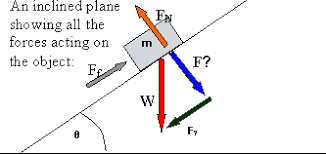## 4ESO FQ English 2018-2019. Unit 3 DYNAMICS (FORCES). Article 5.

Publicado: 22/03/2019 10:02 por easyfisquim en 4ESO FQ (10th gr Science) 2018-2019DYNAMICS.

- You already know what Dynamic is from what you studied before about forces lesson. But you have anyway a reminder here :

+ Dynamics, branch of physical science and subdivision of mechanics that is concerned with the motion of material objects in relation to the physical factors that affect them: force, mass, momentum, energy. Newtons laws of motion are directly related to Dynamics and, even more, they explain it.

- MASS AND WEIGHT :

* Activity 1.32  :   Gravity and weight.                                          ( Monday )

*   These are the questions to answer  looking up the following links :

1.32.a   GRAVITY, MASS AND WEIGHT. ( slides )

1.32.b   MASS AND WEIGHT.     (hyperphysics website with definitions and equations).

1.32.c   GRAVITY.

Check your weight on other planets.

Here you can work out mass, gravity or weight.

When you finish answering all the questions.           ( 20 points ).

+ Do this PROBLEM about finding Jupiters gravity from knowing a persons mass and his/her weight.

> When attraction between Earth and an object is considered, Newtons gravitation equation becomes like this :

+ NEWTONS GRAVITATION EQUATION PROBLEMS.       Just try them !!

+ Here you have a problem about Gravitational attraction force  ( wording pic and then a solution pic ) in
which one you have to solve for another variable different from the attraction force F.

+ At the following website you can check all whatever you want about GRAVITATION !!   ( Keplers laws included ).

+ VIDEO 1  easy explained the 3 Keplers laws.
+ VIDEO 2   easy explained the 3 Keplers laws.
+ VIDEO 3  easy explained the 3 Keplers laws.
+ VIDEO 4  easy explained the 3 Keplers laws.
+ VIDEO 5  even one more video explaining Keplers laws.
+ Right  HERE  you can find a page with an easy way to learn and understand   Keplers laws.

+ Lets try now the exercises 1 and 3 from the following website about Keplers laws.
+ Lets try a few more problems about solving and using Keples laws.

* Activity 1.34  :   Reviewing Mass and gravity.                                          ( Monday )

1.34.a   Pics of earth, gravity and weight to make the poster.         ( 10 points ).

1.34.c   Fun way to review forces.              ( 5 points ).

Copy this outline on a sheet in order to review some of the forces studied.

LINK 2  ( Weight , mass , gravity ).

* Activity 1.33  :   Gravity and weight test.                                     ( Tuesday )

GRAVITY , MASS AND WEIGHT TEST.                                ( 10  points ).

Newtons laws quizzes.

-  Gravity text to be worked on class :  Page 1   Page 2   Page 3   Page 4

-  Gravity cards for a talking activity :

- INCLINED PLANE ( advanced extra class ) :

- An inclined plane, also known as a ramp, is a flat supporting surface tilted at an angle, with one end higher than the other, used as an aid for raising or lowering a load. The inclined plane is one of the six classical simple machines defined by Renaissance scientists.

- Now it is time for you to achieve an exercise like that. Just try doing the
following exercise :

+ Wording exercise 1

Find the components of a force F whose magnitude is 20 N and its direction is
defined by angle θ = 30° made by the force and the positive x-axis as shown below.

+ Wording exercise 2

Find the components of a force whose magnitude is 50 N and its direction is given by                           angle α = 25° made with the negative y-axis as shown in the diagram.

+ You can find the solutions and explanations about the way to achieve
them HERE.

+ HERE   ,   You can find more exercises of the same kind.

Comentarios  Ir a formulario